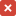1. OMG Issue

# DTV11 — incorrect formula for Gregorian year length

• Key: DTV11-89
• Legacy Issue Number: 19281
• Status: closed
• Source: yahoo.com ( Michael Deckers)
• Summary:

REFERENCXE:
section 11.7, 1st Definition on p 141:
" In mathematical form, the definition
above is:
sd = 685 263 + (365 * y)
+ (y/4) - ((y/100)*2)
+ ((y/400) * 2)
where:
sd is the index of the starting day
y is the index of a Gregorian
year ­ 1601
y >= zero
/ is integer division "

PROBLEM:
The formula is incorrect. For instance,
for years 1700 and 1701 the formula gives
sd(1700) = 685 263 + (365*99)
+ (99/4) - ((99/100)*2)
+ ((99/400)*2)
= 685 263 + 365*99 + 24
= 721 422
sd(1701) = 685 263 + (365·100)
+ (100/4) - ((100/100)·2)
+ ((100/400)·2)
= 685 263 + 365*100 + 25 - 2
= 721 786
implying that the year 1700 had only
364 d, which is obviously incorrect.
The formula gives the wrong year
length of 364 d for all Gregorian
years Y wher Y mod 400 is one
of 100, 200, 300.

PROPOSED CORRECTION:
Omit the incorrect factor 2 twice, and
correct the corresponding note trying
to justify that factor. Many sources
give the correct formula, valid for all
integral year numbers 1601 + y:

sd(1601 + y)
= sd(1601) + (365 * y)
+ floor(y/4) - floor(y/100)
+ floor(y/400)
where:
y is the index of the Gregorian year
with number (1601 + y)
sd(1601 + y) is the index of the
first day of the Gregorian
year (1601 + y)
flooris the largest integer
that is <= x

• Reported: DTV 1.0 — Fri, 7 Mar 2014 05:00 GMT
• Disposition: Resolved — DTV 1.1
• Disposition Summary:

The formula was incorrectly represented. It will be corrected as suggested. The changes below incorporate several changes from Issue 19280 that correct errors resulting from faulty calculation of year lengths and incorrect base values. Two Examples of coordinate computations are revised to use the corrected formula.
The formula for the index origin of the Gregorian months scale is also incorrectly written.

• Updated: Fri, 6 Mar 2015 20:58 GMT